# Game, set, maths (no more tennis puns)

A while ago, my friends and I learned of a brilliant and simple game, played by comedians Alex Horne and Tim Key. We
discovered a clip of them playing the game backstage at a show, and were immediately hooked. It’s a naming game, requiring a vague knowledge of a bunch of famous people, and anyone can play. The game is called No More Women (reasons for which will become clear later) and I’m taking the opportunity both to share the rules of the game with you, and explore a little of the maths behind it.

#### No More Women“Simon Singh, no more authors.”
Richard Cooper, CC BY-SA 3.0

Each move in the game consists of two phases. First, you name a celebrity (for example, “Simon Singh”). Then, you exclude a category of people—and primarily, the category you give must include the person you’ve just named (for example, “no more authors”). Play then passes to the next person, who must name another celebrity—but crucially, they can’t name anyone who falls in a category that’s already been excluded. Play continues until someone’s stated celebrity is proven disallowed, or they can’t think of someone.

There are a few other rules—for example, you’re not allowed to disallow everyone. There must always be at least one person left in the remaining chunk of people that are allowed—for example, having heard the move described previously, you wouldn’t be allowed to say “Courteney Cox; no more people who aren’t authors”—as the two categories, ‘authors’ and ‘non-authors’, together make up the whole set of people.

If the people you’re playing with suspect that your most recent exclusion has made the next move impossible, they may challenge—and you’d have to name someone you can think of who’s still allowed. Challenging like this is a risky move, as you’re then not allowed to say the example just given, and you have to think of yet another one.“Courteney Cox, no more actors.”
Alan Light, CC BY 2.0

It’s also generally agreed that the people you’re naming should be famous people—as in, people everyone might reasonably be expected to have heard of. If you name someone that nobody has ever heard of, your fellow players can insist you think of someone else, or decide
whether a quick cheeky online search is allowed, to verify that they are indeed a tennis player (or whatever you’ve claimed them to be). It’s a matter of opinion whether someone who’s not a real celebrity but is known to everyone in the group playing should be allowed, although this does make it a bit more fun if you allow it.

Also, the properties you give should be ones which people might reasonably be expected to know the truth value of for the majority of people you might name: while it does exclude a sensible proportion of people, “no more June birthdays” is harsh unless you are prepared to let people look up every single name then given to find when their birthday is—and that kind of thing leads to cheating. Impromptu discussion can also break out, for example around what exactly constitutes an author—does it have to be someone who makes a living writing books, or does a presenter who’s written an autobiography count as an author, since they have a published work?“John Venn, no more logicians.”

The title of the game is obviously a nod to one massive move you could make in the game, excluding basically half of all people (although is it half of all famous people? Smash the patriarchy etc). As the game continues, the set of people you’re allowed to name gets
increasingly small, and the game becomes more difficult. The rate of increase in difficulty depends on how cruel your fellow players are with category choices—“no more brunettes” is much harsher than “no more people from Bristol”, but if that’s the only fact you know for sure about your given celeb, you’ll have to go with that.

Particularly mean moves include “no more currently living people” and “no more people whose first and second name begin with different letters”. The trivial move is to say, for example, “Pat Sharp; no more people who used to present the kids’ TV show Fun House”, excluding precisely one celebrity (this is valid, but boring).

This is an excellent game to play when you’re all, for example, sitting around on public transport, or somewhere you don’t have access to conventional game-playing equipment and/or tables. Part of the challenge is remembering all the categories that have been counted out already, although if you prefer you could write down the categories on a board or piece of paper everyone can see as they’re named, and be real sticklers. But is writing down the names in a list the best way?

#### No more lists

A more interesting way of writing down the moves could make the game more concrete and help us understand it mathematically. Since each move divides the space cleanly (assuming the definition of your category is precise enough), you could imagine the people all on a page, then draw a circle around a set of people and everyone inside the circle could be in the category, and vice versa. This leads us to a natural way to describe moves in the game—using Venn diagrams.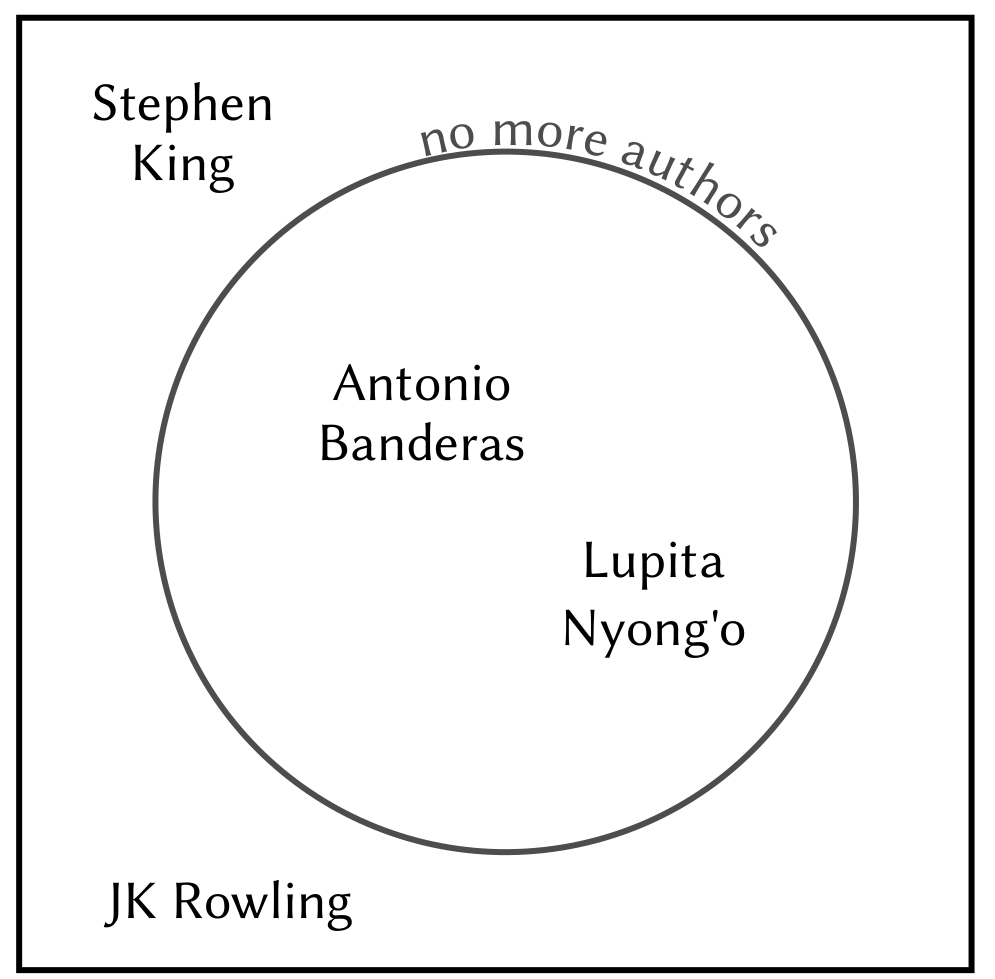Everybody knows Venn diagrams—the overlapping circles of categories described by John Venn in 1880, now a staple of set theory and a lovely way to visualise sets of things with certain properties. In this game, by definition, every person falls neatly in or out of a set. For example, you could have “no more authors” which would exclude everyone inside the set ‘authors’ . In terms of Venn diagrams, the nicest way to display this would be to call the outside of the circle ‘authors’ and the inside ‘non-authors’, so the circle contains all the people still allowed (see diagram). By our rules, there must be at least one person outside the circle (in particular, the one you just named on your turn), and one person inside the circle (which you’d need to be able to name if challenged).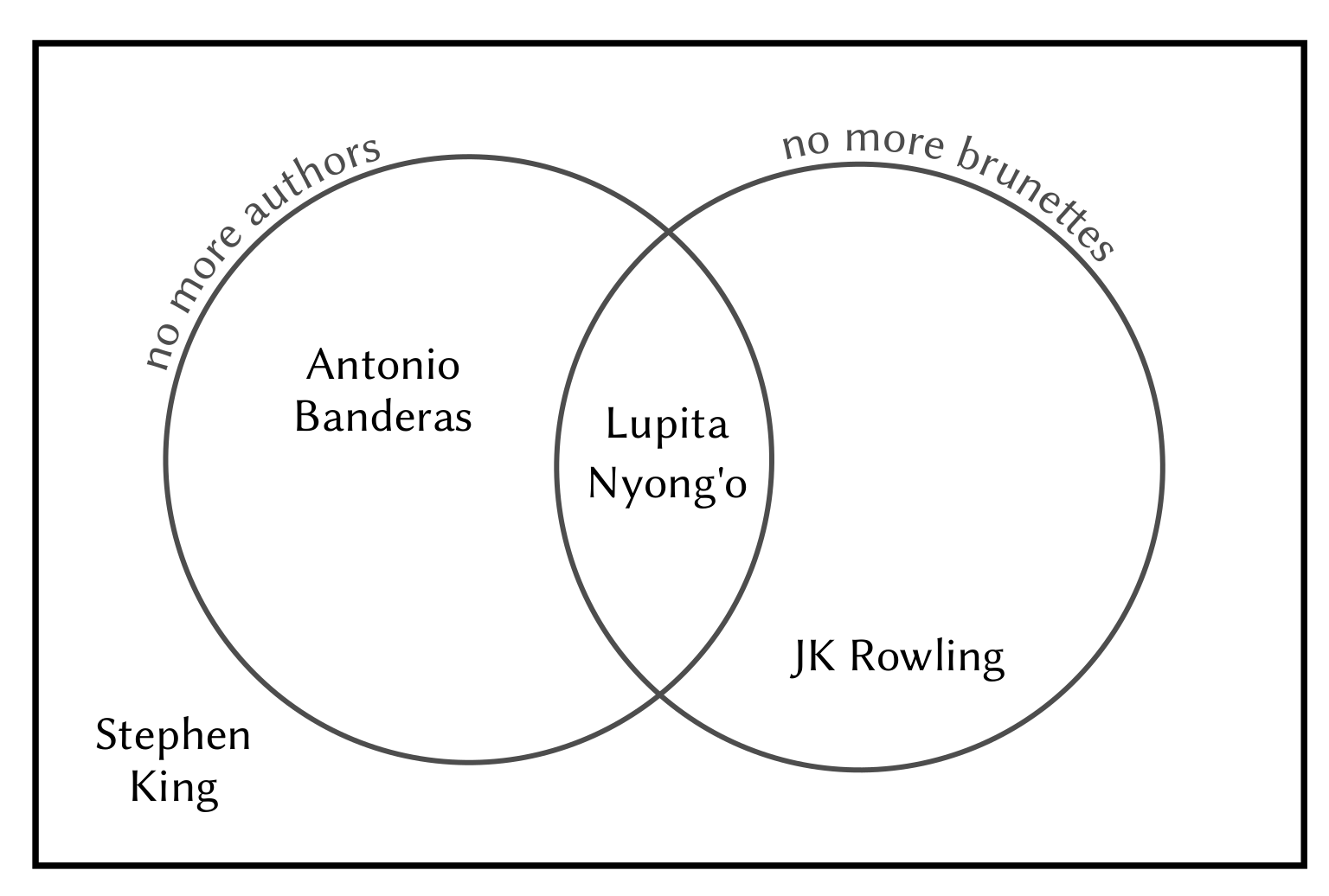A second move (eg “no more brunettes”—agreement will have to be reached whether you count natural brunettes only) could then overlap with this, restricting the next move to those in the intersection of those two circles. You’d also need at least one person to be in the region you’d just excluded (the non-author brunette you just named) and at least one still allowed (one non-author non-brunette you can name if challenged). However, it’s not necessary for there to be a non-brunette author (no offence, JK Rowling), as that category has already been excluded, so we don’t care what happens there.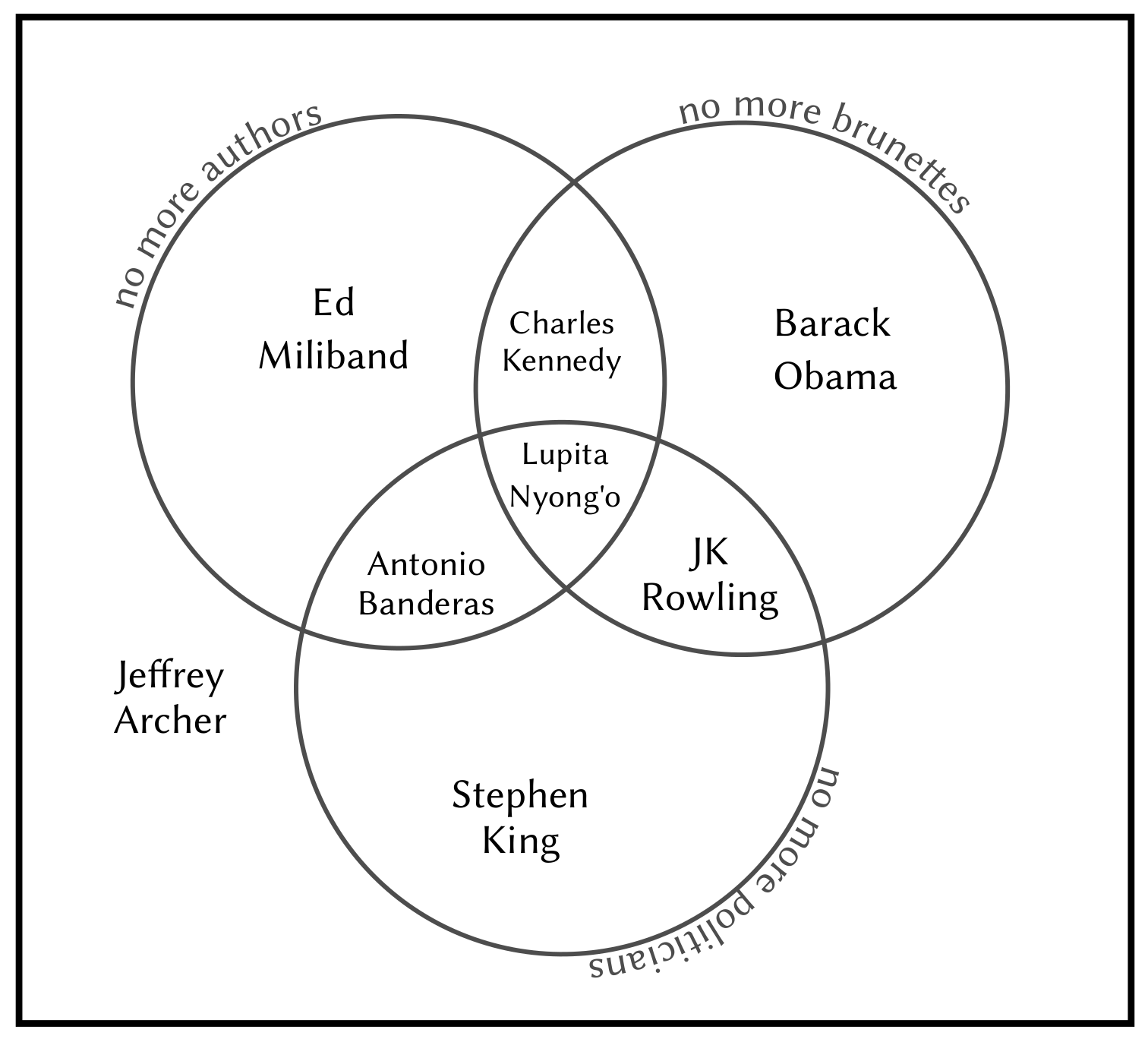Each move of the game adds another circle to this diagram—it’s easy enough to draw a third circle to create a three-way Venn diagram. Let’s say, for example, someone says “no more politicians”; this would overlap with all four existing categories (brunette authors, in the outer region; brunette non-authors; non-brunette authors; and non-brunette non-authors, which is where allowable moves still lie). You’d need to have named a non-brunette non-author politician as your turn (we went with Charles Kennedy, confirmed redhead) and you should also be able to name a non-brunette non-author non-politician (such as Lupita Nyong’o, who was in The Jungle Book, but hasn’t published one yet).

A four-way Venn diagram using only circles is not possible—in the example shown on the left below, not all sets of intersections are present. For example, there would be nowhere to place Steven Tyler (a brunette non-author who’s tall and not a politician). However, a four-way diagram can be constructed using ellipses (shown on the right), and was designed by John Venn himself.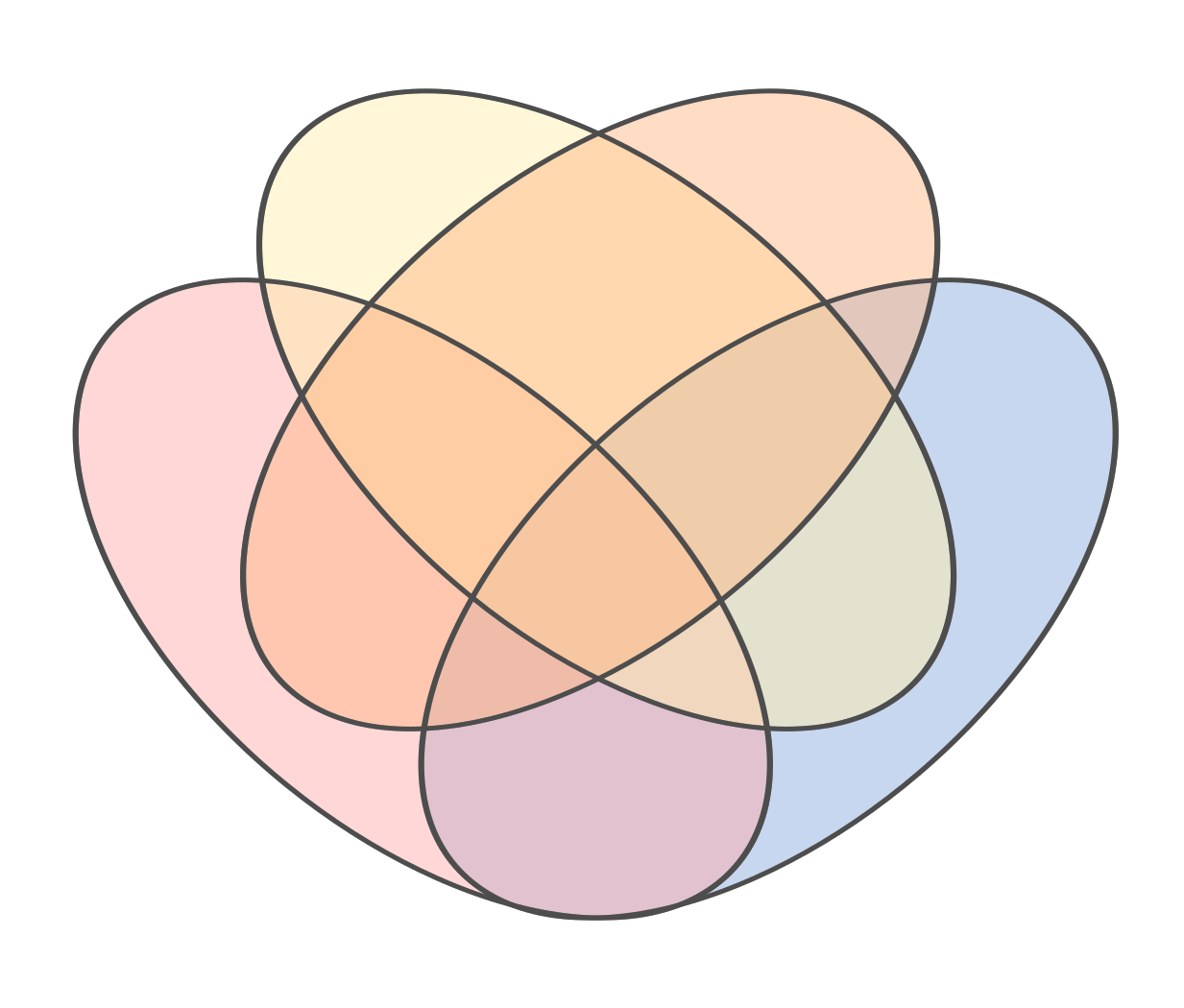Two attempts to draw a four-way Venn diagram. In the attempt on the left, you can dream on if you think there’s a space for Steven Tyler.

It is also possible to construct Venn diagrams with five identical pieces—the one on the left, devised by Branko Grünbaum has rotational symmetry. The five-way Venn diagram shown next to it is called an Edwards–Venn diagram, and was devised by Anthony Edwards. They represent a way to create Venn diagrams with arbitrarily many regions, using sections of a sphere projected back down onto a plane, and were devised while designing a stained-glass window in memory of Venn. Starting with the left and top hemispheres, given by the two rectangles, and the front hemisphere represented by the circle, the next set is the shape made by the seam on a tennis ball (winding up and down around the equator) and further sets are made by doubling the number of oscillations on subsequent winding lines. They’re sometimes called cogwheel diagrams, due to the shape.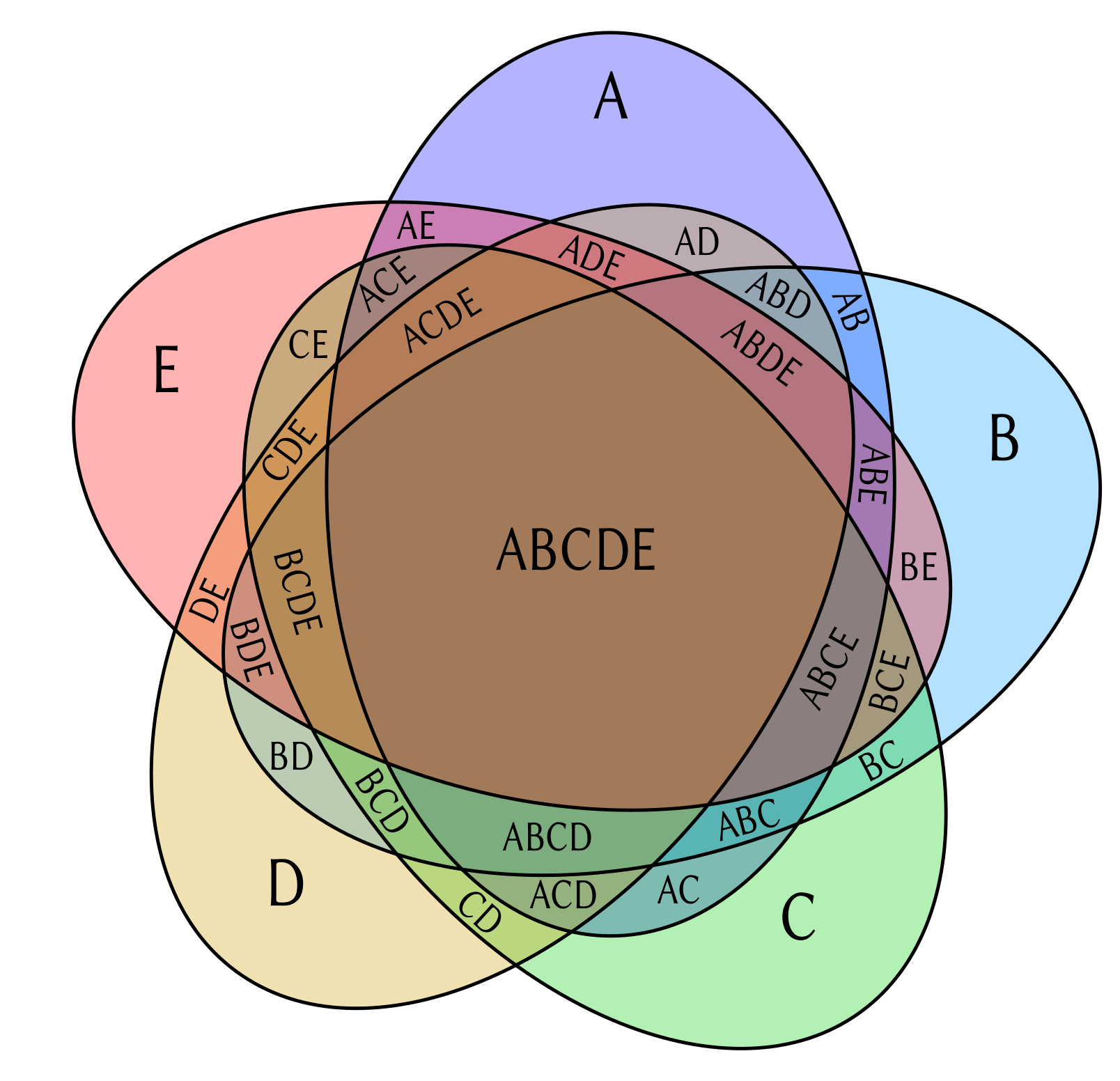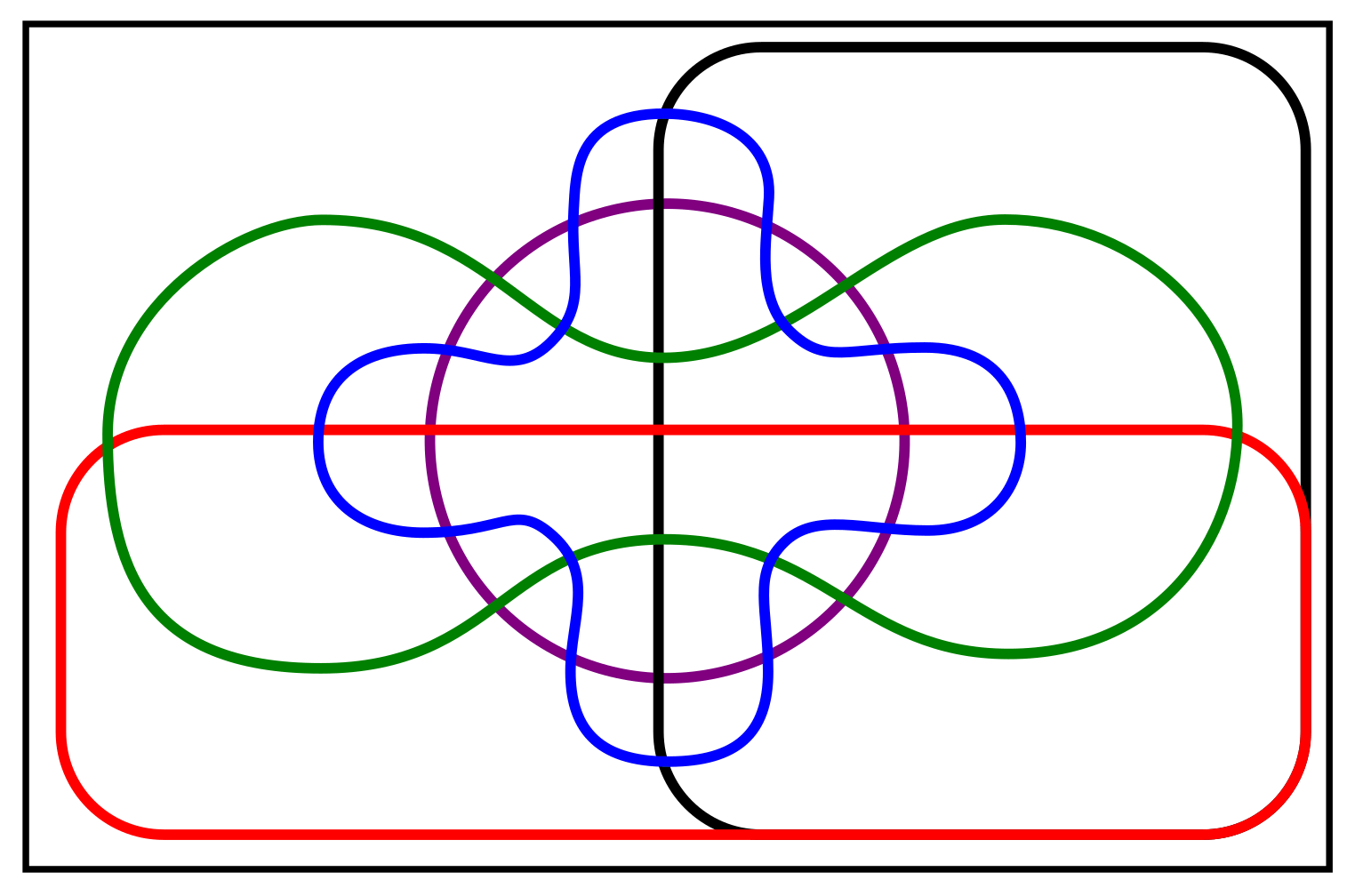Two five-way diagrams: Grünbaum’s diagram (left) and an Edwards–Venn diagram (right)

Technically any number of segments is possible, although the diagrams get much more complex as you go on. There’s a lovely interactive seven-way Venn diagram by Santiago Ortiz (on his website: moebio.com/research/sevensets—neither he nor I am able to determine the original author of this shape). Of course, this would still only get you seven moves into a game of No More Women, and representing a full game in diagram form would be challenging and likely uninformative.

#### No more Venn diagrams

Another way to interpret the game’s structure would be to use half-planes. If you could theoretically arrange everyone in the (so far useful) 2D plane of all celebrities—presumably a private jet—then a category excluded could be represented by a line cutting the plane in half, with all the allowed persons on one side and the disallowed persons on the other side.A goat acting out a maths puzzle.

For example, the line $x=2$ describes a vertical line on an $x y$-plane running through the point $2$ on the $x$-axis, and everyone to the left of the line might be an author and everyone to the right not an author. Then, a second line at $x + y = 1$ might cut diagonally across, with brunettes above and non-brunettes below. It reminds me slightly of the loci problems we used to play with at school—a way to visualise solutions to sets of linear equations, or to determine which bits of a field a tethered goat can reach (for some reason, it’s always a goat).

We can intersect arbitrarily many half-planes to define the space of allowed people. In fact, any convex set can be described as an intersection of half-planes. But this is probably not useful either—firstly, the sections will become arbitrarily tiny and hard to see,
much as in the Venn diagram case; secondly, you’re kind of imagining the people all standing in the room (or on a very, very big plane) and each time you define a new line you’d need everyone to be miraculously standing on the correct side of it. This is leading me to imagine celebrities looking upwards, then sprinting across so they’re on the correct side before a giant imaginary looming line comes crashing down, which is fun to picture, but not hugely helpful. And finally, the lines are defined in a pretty arbitrary way—the categories we’re using are not quantitative (unless you’re using a category like “no more under-25s”, in which case you can plot that on an axis), and otherwise there’s no natural way to assign an equation to a category, so it’s a bit unsatisfying.

#### No more diagrams

So I guess we’ll have to fall back on classic set theory. Developed by Cantor, while he was attempting to work out a way to compare the magnitude of different infinite sets, the theory of sets underpins a huge amount of mathematical rigour and thinking, and contains parallels with algebra and logic in ways that illustrate the beauty of mathematics in its purest form. But we just want to play a stupid game about famous people, so here goes.

In set notation, we might define:

$$\text{Authors} = \{ \text{Simon Singh}, \text{Stephen King}, \text{JK Rowling}, … \}$$

The set is specified by the list of things in brackets, so this set equals this collection of people. Then our game would consist of moves as follows:

$$\text{Simon Singh}\in\text{Authors}$$

$$\overline{\text{Authors}} \neq \varnothing$$

Here the $\in$ symbol means ‘is an element of the set’. We use a line above the name of the set to mean ‘the complement of this set’, or the set of all things in the universe that aren’t in this set. In the Venn diagrams we defined earlier, $\overline{\text{Authors}}$ would be the inside of the ‘no more authors’ circle, and the set $\text{Authors}$ would be everything outside this circle.

We’ve also specified, in the second line, that the set $\overline{\text{Authors}}$—the set of all non-authors—is not equal to $\varnothing$, where this symbol denotes the empty set. This means that set is not empty, because something exists in it.

Play continues:
$$\text{Courteney Cox}\in\text{Brunettes}\cap \overline{\text{Authors}}$$

$$\overline{\text{Brunettes}} \cap \overline{\text{Authors}} \neq \varnothing$$
Here we’ve used the $\cap$ symbol to define an intersection—this is the set of all things that occur in both the given sets—here, brunettes who are also not authors.

Along with the $\cup$ symbol for a union (the set of all things in either or both sets), this is one of the two main operations you can do with sets, and they correspond respectively to the AND and OR operators in Boolean logic, in a rough sense. On the Venn diagram, the intersection is the part of those two sets which overlaps—here, Brunettes is the outside of one circle, and $\overline{\text{Authors}}$ is the inside of another circle, so this is the portion of the non-Authors circle that doesn’t overlap with the non-Brunettes circle.

Again, the requirement of being able to name an example means that the set of non-brunette non-authors now has to definitely not be empty, and must contain something.

$$\text{Ed Miliband}\in\text{Politicians}\cap \overline{\text{Brunettes}} \cap \overline{\text{Authors}}$$

$$\overline{\text{Politicians}} \cap \overline{\text{Brunettes}} \cap \overline{\text{Authors}} \neq \varnothing$$
And so on. Sets can intersect arbitrarily, and while this doesn’t necessarily give us a nice visual way to imagine the celebrities, it
does give us a formal structure. Properties of set intersections can be considered as they apply to the game—for example, set
intersection is commutative:
$$A \cap B = B \cap A.$$
This means it’s independent of ordering, which feels obvious—brown-haired women are women with brown hair, duh—but in mathematics you have to be careful whether the order in which you do things matters (multiplying by three then adding four is different to adding four then multiplying by three, and often on Facebook only a real genius can work out the correct answer to this math problem, so keep your wits about you).

Intersection of sets is also associative:
$$A \cap B \cap C = (A \cap B) \cap C = A \cap (B \cap C).$$
Intersecting three sets is the same as first intersecting two of them, then intersecting the result with the third one. Because the action of intersecting sets is associative, it doesn’t matter which order you do this in, you’ll get the same result. Someone who’s a brunette author and also a philanthropist could also be considered to be an author/philanthropist with brown hair.

These two properties together mean that if you’re playing the game, and the categories defined are given in a different order—say, you’re playing against two others and they each give a specific category—they could occur in either order and still leave you with the same challenge afterwards. Non-brunette non-authors are just as hard to think of, and just as numerous, as non-author non-brunettes. It will, however, affect the choice of named celebrity each of the two other players is allowed to give.

There are other properties of sets you could think about in terms of gameplay—for example:

$$\overline{A \cup B} = \overline{A} \cap \overline{B}.$$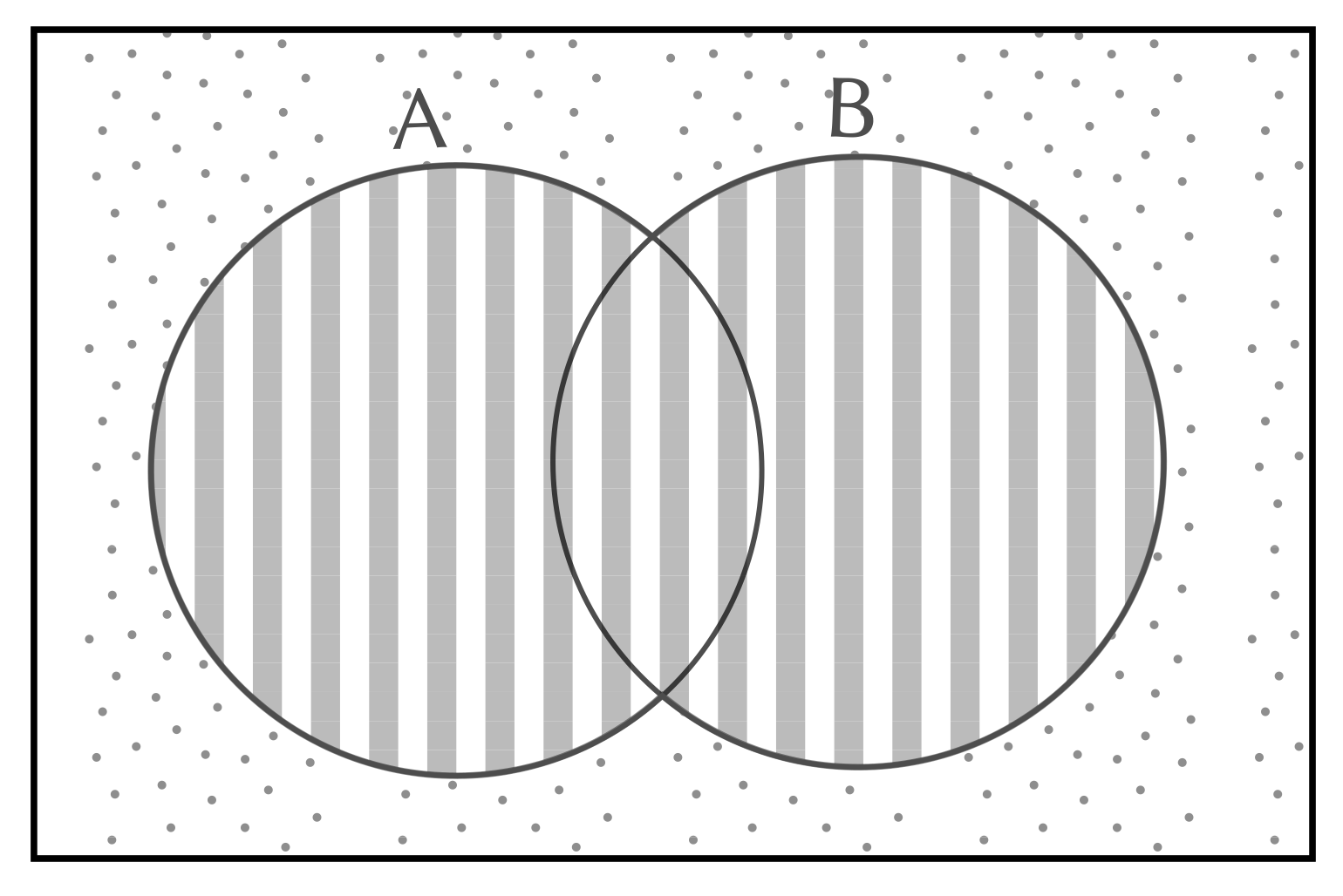This says, the complement of the union of two sets is the same as the intersection of the complements. This is shown in Venn form to the right,
and it essentially means that if you consider the complement of each set (the things outside it) individually, the things they have in common will be the same as just the things that are in the complement of the union of these two sets—consider them together as an overlapping shape, and look outside of that.

In the context of the game, you can consider this to mean that if you’re looking for someone who’s not a brunette AND not an author (because of two successive turns that have occurred in the game), you need to think about the set of people who are either a brunette OR an author, and look for someone not in that set. Again, this feels obvious when you think about it in the context of naming celebrities, but the set theory confirms it.

Maybe formalising the game in this way will help you to get your head around the ideas of sets and set theory, if you’ve only recently encountered it. As someone who studied it—cough—years ago, it’s become part of my way of picturing any kind of problem like this, and a natural language with which to describe intersecting sets. I’ve been trained through years of maths to take any fun thing and abstract it into some symbols on a page. Yay!

#### No more No More Women

Of course, the mathematician’s real job is abstraction then generalisation—so this game could be played using any well-known set of things that can be categorised according to properties, and we have piloted among ourselves a much more niche version of the game, provisionally titled No More Integers

Played on the set of all numbers, starting with the complex plane, it’s basically a free-for-all and in general we’ve found it becomes very difficult very quickly, not just to name a number that hasn’t been excluded, but to think of a category that works and doesn’t just make the game totally boring and impossible. If non-integers are excluded early on, and then numbers with given factors start getting thrown out, it can get a bit like the coding/drinking/coding-while-drinking game Fizz Buzz.

It’s also totally possible for someone to say something that’s wrong/has already been excluded but nobody notices because their brains are all fried from factorising numbers in their head. It might take a few plays through before you all learn the best way not to end up in a mass brawl with whomever excluded the primes, but hopefully you can find some enjoyment in it. Or just play the version with celebrities—it’s a lot of fun.# Thinking outside outside the box

There aren’t many puzzles that became famous as a corporate cliche, but the nine dots problem is certainly one of them.

There are nine dots arranged in a square, and your challenge is to join all the dots using only four straight line strokes of a pen, and with your pen never leaving the paper. No tricks are needed, no folding the paper and no using an ultra-fat pen. You’ll have no problem finding a solution with five lines, but in the unlikely event that you’ve never seen this puzzle before, four lines might prove to be a struggle.

The puzzle first rose to notoriety when Sam Loyd included it in his 1914 cyclopedia of puzzles. He called it the Columbus egg puzzle, after an apocryphal story about Christopher Columbus challenging his colleagues to get a boiled egg to stand on its end — a challenge that seemed impossible until Columbus crushed the base of the egg and rested it on its now flat bottom. Easy when you know how.

The solution to the nine dot puzzle led to the cliche of ‘thinking outside the box’ (that’s a massive clue to the solution, but if you still can’t find the answer, you’ll find it at the bottom of the article). The solution is a useful metaphor for the challenge of problem solving, when we often become restricted by constraints of our own making. Deliberately challenging your assumptions and allowing yourself to be ‘silly’, at least for a short period, can be a handy strategy for creative problem solving in all walks of life.

What I find interesting, however, is that the lessons of the nine dots puzzle are often quickly forgotten when the puzzle is modified.# Baking a Menger sponge sponge

If you’ve been following Chalkdust for a while, you’re probably already aware of the MathsJam gathering. You might even have been yourself. For those who aren’t aware, it is an annual event where maths lovers get together to talk, play, sing and generally have fun with maths. One feature is a baking competition: attendees bring a maths-related cake and there are prizes for the prettiest cake, the tastiest cake and the best maths in a cake. This is the story of one of those cakes: the diagonal cross-sections of a Menger sponge.

#### Why a Menger sponge?

I was looking around for a cake subject, and had considered a famous fractal known as the Menger sponge. Then I came across an article that showed what it looks like when you make a diagonal cut through the middle. It was totally unexpected! I decided then and there that I had to make this cake.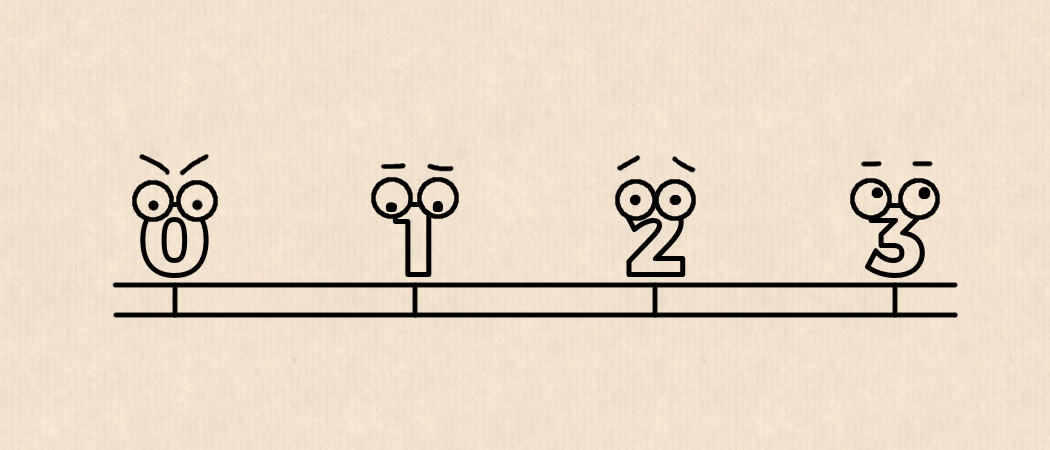# e to thee xI’m e,
If you don’t know me
I live
Between 2 and 3.

This is my story.

I’m misunderstood.
Everyone thinks I’m just 2.71
But there is more to me
That they don’t see
I wish they would.

And I’m named after a letter
Which makes things worse!
The other numbers laugh at me for that too.

So I say to them—look at the things I can do,
I mean, surely I’m the only number
To have walked this earth
And expressed themselves in verse?

But that’s not cool, that’s not fashionable,
It’s all about being able to
Express yourself as something fractional, instead.

Otherwise they say there is something wrong with you
You’re crazy,
Irrational.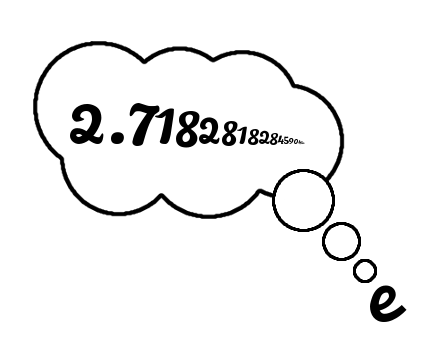Natural numbers can count themselves
Lucky.
They can easily find their space
Because our place
On that line
Is defined
By our digits
And I don’t know all of mine.

But there was a time
When there were three of us who didn’t fit.
Pi also didn’t know,
Exactly where to stand, or sit,
And next was i,
She was in a dimension of her own!

I thought the three of us
Were meant to be,
We were all part of the same identity.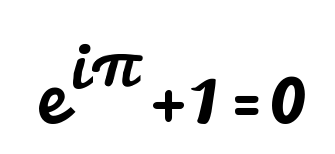But then we grew up.

I started to see Pi from a new angle,
I loved that rest of her body was basically a rectangle.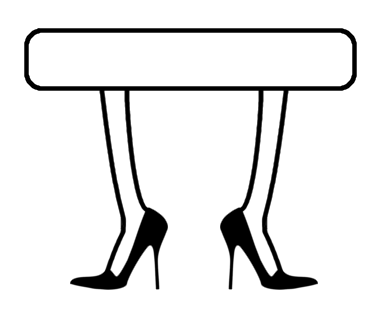We went on a couple of dates.

But then came Pi Day:
Three, Fourteen.
She let them approximate her!And she became
An overnight sensation,
A household name,
One of those faces
Everyone knows.

And lost in that world of meaningless approximation
She wouldn’t let me take her to more than two places.

Time passed,
And even my old friend i and I grew apart,
Of age,
I began to see what others had told me
And I’d refused to believe:
i was imaginary!You might be wondering what about Tau?
She is twice the number Pi will ever be,
But Pi and Tau they are similar
And for me it was all a bit familiar,
Tau, she’s just too Pi.So that’s it, back to lonely me.
It was hard
And I’m not a negative number.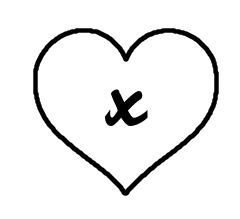But then
I met her,
$x$.

She said ‘I’m $x$’,
I asked, ‘are you a multiplication sign?’
She laughed, ‘I get that all the time’,
‘No, I’m curly $x$,’
She said.

She’s curly $x$, she’s sort of curvy $x$,
But that’s not it,
I’m not one to make judgements
Based on digits or figures,
She’s different,
She’s fun.

And it’s true
I can’t always work her out
But I like that, too.
With her is where I always want to be,
I want her to be my unknown quantity!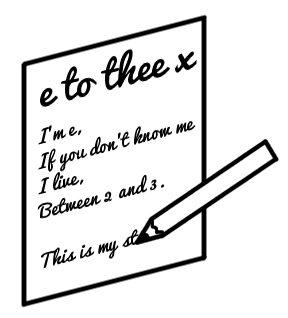So I wrote her a poem
‘e to thee $x$’,
(It was better than this).

She opened it, tentatively,
Aloud,
Other numbers could hear!
Never have I wanted so much
To just
Disappear.e to thee $x$, this poem I’d written,
This part of myself I’d given
Was supposed to feel just right,
But it was the opposite
It was the inverse of natural
(Logarithm?)Actually, that poem, didn’t happen.
I just dreamt that.
It’s the 21st Century
I sent her a Snapchat.

I sent it and then screamed inside
Until she read it, and she replied.

I won’t tell you what she said
But the bit that is etched in my head
Is the final line, a string of Xs.

The first was curly $x$,
That’s her name,
The rest were to say
She’d like to see me again.

And if you’re worried that what is essentially
Just did something unexpected to your heart,
Then shame on you!
Numbers can have feelings too.

So this story is about me and $x$
And the number she’s shown me I can be,
I’m e, I live between 2 and 3,
I don’t know where exactly
I don’t care!

With $x$, I see things from a different view,
I laugh in the face
Of the quite frankly ridiculous number queue.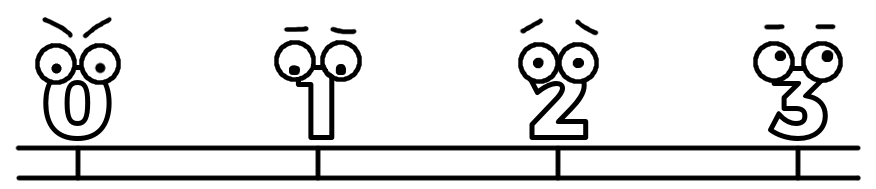I’m me, I’m e!“I’m really sorry, the vampirograph indicated that you are a vampire.” Imagine that you (or your mother/brother/girlfriend/pet scorpion) received such a message. You’re probably terrified, worrying about the future, thinking about the upcoming treatment. Wait a moment! Before you start panicking, consult… a mathematician.

Medical tests aren’t perfect. Testing positive for an illness doesn’t necessarily mean that you’re sick; for many reasons, tests can detect things that aren’t really there. On the other hand, a negative test result doesn’t exclude the disease without any doubt. The question is: can we quantify the level of uncertainty linked to a particular test? Or in this case: if you test positive on a vampirograph, what’s the probability that you’re really a vampire?

A similar question was tackled in the 18th century by a Presbyterian minister, Reverend Thomas Bayes. Bayes’ theorem became a basis for statistical inference, even though conclusions drawn from it are sometimes counter intuitive. His result gives us an explicit formula to update our prior belief about the probability of some event of interest based on additional evidence:
$$\mathbb{P}(\text{event}|\text{evidence})=\mathbb{P}(\text{event})\frac{\mathbb{P}(\text{evidence}|\text{event})}{\mathbb{P}(\text{evidence})}.$$Let’s get some intuition about this equation. I assume you’re familiar with the notion of the probability measure $\mathbb{P}$; don’t worry about a rigorous definition, a common interpretation—ie how likely the event is—will suffice. The mysterious symbols $\mathbb{P}\left(\text{something}|\text{something else}\right)$ denote a conditional probability—how likely $\text{something}$ is given that $\text{something else}$ happened.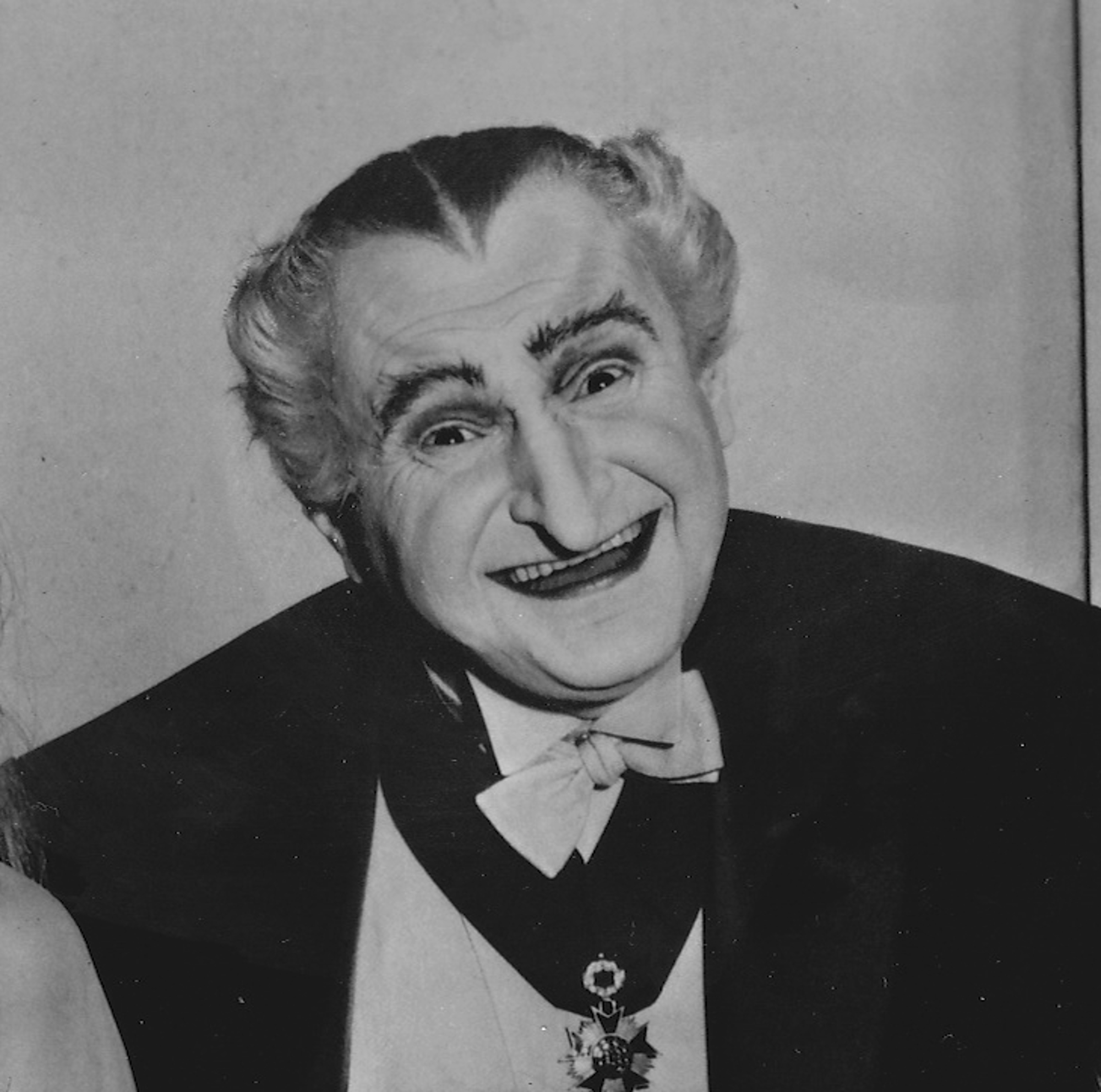$\mathbb{P}(\text{this picture contains a vampire}) = 1$

Now we’re ready to take a look at Bayes’ theorem again. In the beginning we have some vague idea about the probability of the event, a so-called prior probability $\mathbb{P}(\text{event})$. For example, let’s say we’re interested in the probability that this cute guy we just met is a nice person. We might base our estimation on our previous experience with similar people or the fact that he’s a mathematician (mathematicians are usually nice people, aren’t we?), but our knowledge is pretty limited. However, later we gather some additional observations (he smiles a lot, he helps us solve a ridiculously difficult equation etc) and we keep updating our prior probability. In the end we’re left with a posterior probability $\mathbb{P}(\text{event}|\text{evidence})$ of him being a nice person given the evidence we have.

Hold on, how did we get from vampires to estimating if a cute guy is also nice? (No, I’m not a Twilight fan.) Bayes’ theorem has many applications! Before we approach our vampire problem, we need to make a few assumptions—all numbers come from my imagination [citation needed]. The scenario is as follows:

• Of the vampirography participants, approximately 2% are in fact vampires.
• When someone is a vampire, they have 0.85 chance of being detected, ie getting a positive result from a vampirograph (so there is a 0.15 chance they remain undetected).
• When someone is an actual human being, they have 0.1 chance of being falsely “detected” (so the remaining 90% of vampirographs give legitimate negative results).

In other words numbers:

 vampires (0.02) humans (0.98) positive result 0.85 0.1 negative result 0.15 0.9

Now assume that you tested positive on a vampirograph. What are the chances that you’re a genuine vampire? Time to ask Bayes for help.

We’re interested in $\mathbb{P}(\text{vampire}|\text{positive result})$—the probability that you’re a vampire if you tested positive. So what do the numbers tell us?

• The probability that you’re a vampire based only on the fact that you’re getting a vampirography: $\mathbb{P}(\text{vampire})=0.02$.
• The probability that you’re a human based only on the fact that you’re getting a vampirography: $\mathbb{P}(\text{human})=0.98$.
• The probability that you test positive if you’re a vampire: $\mathbb{P}(\text{positive result}|\text{vampire})=0.85$.
• The probability that you test positive if you’re a human: $\mathbb{P}(\text{positive result}|\text{human})=0.1$.

We also need the probability that you test positive regardless of what you are (you’re either a vampire or a human, we assume no other possibilities). This is a bit more tricky, but let’s see what we can squeeze out of our data. We’ll need the law of total probability, which might be interpreted as a weighted average of probabilities, where we average over all possible cases. In our example we have only two possibilities—someone is either a vampire or a human. A vampirograph gives a positive result, in each of these cases: rightly when we deal with an actual vampire and falsely when the participant is human. Therefore we can split our calculation of the probability of the positive result into these two separate cases.
\begin{align*}
\\&=0.85\times 0.02 + 0.1\times 0.98\\&=0.115,
\end{align*}where we have used the law of total probability. Now we’re ready to plug everything into Bayes’ formula:
\begin{align*}
\mathbb{P}(\text{vampire}|\text{positive result})&=\mathbb{P}(\text{vampire})\frac{\mathbb{P}(\text{positive result}|\text{vampire})}{\mathbb{P}(\text{positive result})}\\&=0.02 \cdot \frac{0.85}{0.115}=0.148.
\end{align*}Yes, even though vampirography seems to be pretty good at detecting vampires, if you test positive the chance that you’re actually a vampire is only 14.8%! No need to panic yet, I guess. Why is this number so small though? This is always the case with very rare conditions, when the prior probability has a big influence on the posterior. Before the test, the chance that a randomly chosen participant prefers human blood to ketchup is very small, only 2%, because this is the proportion of vampires in the population. Getting a positive result significantly increases this value, but we started from a low level, so the final probability remains quite low. Luckily most dangerous diseases, such as different types of cancer, tuberculosis or AIDS, are relatively rare, which means that conclusions of our study would be similar if you replaced being a vampire with a real illness. This means that a worrying test result was most likely a mistake, not a real problem, and that you should follow up with a doctor and possibly repeat the test.

Conclusion? Take care of yourself and get tested regularly (this article isn’t sponsored by the NHS in case you’re wondering). However, if you test positive, don’t panic and consult with a doctor… or a clergyman. Preferably Thomas Bayes.# Counting palindromes

About a year ago, I was walking to work when I started to think about palindromes. This was partly because I’d seen one at work the day before, but mostly because my iPod had packed up on me, leaving me with a good half an hour with nothing better to do.

A palindrome is a word like LEVEL, which reads the same forwards and backwards. Specifically, I was thinking about 5-digit numbers, so my palindromes were things like 01410. I’d seen one like this at work the previous day, which got me thinking-what were the chances of that happening? This turns out not to be a very interesting question to ask—for a random 5-digit number, the answer is simply 1 in 100. The first 3 digits can be whatever they like, and then the remaining two must match up with a 1-in-10 chance for each.# Routes: Edsger Dijkstra

In a 2001 interview with Philip L Frana for the Charles Babbage Institute, Edsger Dijkstra succinctly demonstrated the importance of his shortest path algorithm:

Dijkstra: If, these days, you want to go from here to there and you have a car with a GPS and a screen, it can give you the shortest way from your hotel to Robbie Creek Cove. Yes?
Frana: I did generate a map, yes, on line.
Dijkstra: Did you use it?
Frana: Yes, I did use it.
Dijkstra: Then you used my algorithm this morning.

The roots of mathematics reach back through time for centuries, providing a solid foundation by anchoring our knowledge in the ages of Pythagoras, Euclid, Fibonacci, Galileo, and Newton. However, some areas of mathematics are new. Their roots are not deep, yet they still carry the huge weight of technological advances that we use, and take for granted, every day.# On the cover: Chladni figures of a square drum

The 18th century—the age of enlightenment. Ernst Chladni travelled around Europe demonstrating his ‘musical curiosities’. The star attraction was a novel technique to expose the various modes of vibration of a rigid surface.# Significant figures: Sir Christopher Zeeman

At the Chalkdust issue 06 launch party, we brought along a challenge for our guests: we connected two people up with ropes and challenged them to separate themselves. To make things interesting, they weren’t allowed to remove the ropes from their hands, cut the ropes or untie the knots. Although the trick is 250 years old, it was made popular by Sir Erik Christopher Zeeman who used it as an interactive way to demonstrate topology, hence the challenge became known as Zeeman’s ropes.

Erik Christopher Zeeman was born in 1925 in Japan to a Danish father, Christian Zeeman, and a British mother, Christine Bushell. A year after his birth they moved to England. Zeeman was educated at Christ’s Hospital, an independent boarding school in Horsham, West Sussex. He did not enjoy the experience, feeling it was a prison in which he lost his self-esteem.

In 1943–1947, Zeeman served in the RAF as a flying officer. In his own words:

“I was a navigator on bombers, trained for the Japanese theatre, but that was cancelled because they dropped the atomic bomb a week before we were due to fly out. Since the death rate was 60% in that theatre it probably saved my life, but at the time I was disappointed not to see action, although relieved not to have to bomb Japan, the land of my birth.”

During his service, Zeeman forgot much of his school maths. But this didn’t stop him from going on to study maths at Christ’s College, Cambridge, where he earned his MA.

Zeeman stayed on in Cambridge for his PhD, in which he wrestled with unknotting spheres in 5 dimensions, spinning knots in 4 dimensions, as well as trying (and failing) to solve the Poincaré conjecture (which would only be resolved in 2005 by Gregori Perelman). He was supervised by Shaun Wylie, who had worked with Alan Turing at Bletchley Park during the war on projects including deciphering a German teleprint cipher called Tunny.

## Founding Warwick

In 1963, Zeeman was invited to join the newly established University of Warwick as the foundation professor of mathematics. He initially declined, since he believed Cambridge to be “the centre of the mathematical world”. However, after “a sleepless night”, Zeeman changed his mind and made the biggest move of his life in 1964.

At Warwick, Zeeman was determined to “combine the flexibility of options that are common in most American universities, with the kind of tutorial care to be found in Oxford and Cambridge”. Initially, he recruited lecturers in three main branches of mathematics: analysis, algebra and topology. Legend has it that those he invited to Warwick all declined their offer; his response was to encourage them by telling them that all the others had accepted his invitation. Later on, Zeeman also appointed six lecturers in applied mathematics.

His leadership style was informal, which helped produce an atmosphere in which mathematical research flourished. By the time Warwick accepted its first students in October 1965, the department was already competing with other universities at an international level. The glass building it is now housed in is named after Zeeman in honour of his tremendous effort in founding the department.

Zeeman left Warwick in 1988, and was made an honorary professor there upon his departure. He moved on to become the principal of Hertford College, Oxford and Gresham professor of geometry at Gresham College, London. He retired from these two positions in 1995 and 1994 respectively.

## Catastrophe

From 1966 to 1967, Zeeman was a visiting professor at the University of California, Berkeley. Shortly after his return to Warwick, a dynamical systems symposium was held, attended by many of the world leaders in dynamical systems, including Stephen Smale and René Thom. They inspired his change of discipline from topology to dynamical systems, and prompted Zeeman to spend a sabbatical with Thom in Paris, where he grew fascinated with what came to be known as catastrophe theory.

He is famous for inventing a catastrophe machine, consisting of a circular disc that can rotate freely about its centre, and two elastic bands of identical length attached on the edge of the disc  The other end of one piece of elastic is fixed, while that of the second elastic is free to move on the plane. Zeeman’s machine has some surprising behaviour: as the free end moves around, the disc would do something unexpected: it flips to a drastically different position. The flipping action is a vivid example of a catastrophe: a discontinuous effect resulting from a continuous change of forces. You can plot the set of points at which the disc flips, called the bifurcation set, which takes on a diamond-like shape consisting of four concave edges and four cusps.

According to Hirsch, Zeeman once tried to take his machine with him to the USA. As soon as he mentioned the name of his machine, US customs cleared the room and had Zeeman arrested!

Zeeman played a huge role in making catastrophe theory a hot topic in the 70s. He was keen to apply it in numerous contexts such as nerve impulses, the collapse of bridges, stock markets and even prison riots. On returning to Warwick, he taught a course in catastrophe theory for undergraduates, which soon became extremely popular.

## Outreach and the Royal Institution

Zeeman was not only passionate about his research, he was also heavily engaged in promoting mathematics to the general public. He was the first mathematician to present the Royal Institution Christmas lectures, in 1978. Going by the title of Mathematics into pictures and including a mix of pure and applied mathematics, Zeeman inspired his live audience with the aid of various demonstrations, including his own catastrophe machine. His lectures sparked plenty of enthusiasm; among the live audience was Marcus du Sautoy, a budding young mathematician who would go on to deliver his own Christmas lectures in 2006.

But that was not the only result from Zeeman’s Christmas lectures—they also served as the inspiration behind the Royal Institution masterclasses for both mathematics and engineering. Starting from 1981, the masterclasses were designed to inspire keen schoolchildren across the UK. When I attended them as a schoolgirl in 2005, I had no idea about the history behind the masterclasses at the time!

## Awards and positions

In 1975, Zeeman was elected a fellow of the Royal Society and was president of the London Mathematical Society (LMS) from 1986 to 1988. He also took up many other positions and received various awards—too many to list in one article.

Zeeman was knighted in 1991 for his “mathematical excellence and service to British mathematics and mathematics education”. More recently, the Institute of Mathematics and its Applications (IMA) and the LMS jointly set up the Zeeman medal in his honour to recognise those who “have excelled in promoting mathematics and engaging with the general public”. And you don’t have to be a seasoned professor to earn the medal—the 2016 Zeeman medal was won by author Rob Eastaway.

## Epilogue

Zeeman had three daughters and three sons from two marriages. One of his daughters, Mary Lou Zeeman, became a mathematician herself, eventually collaborating with her father in mathematical ecology.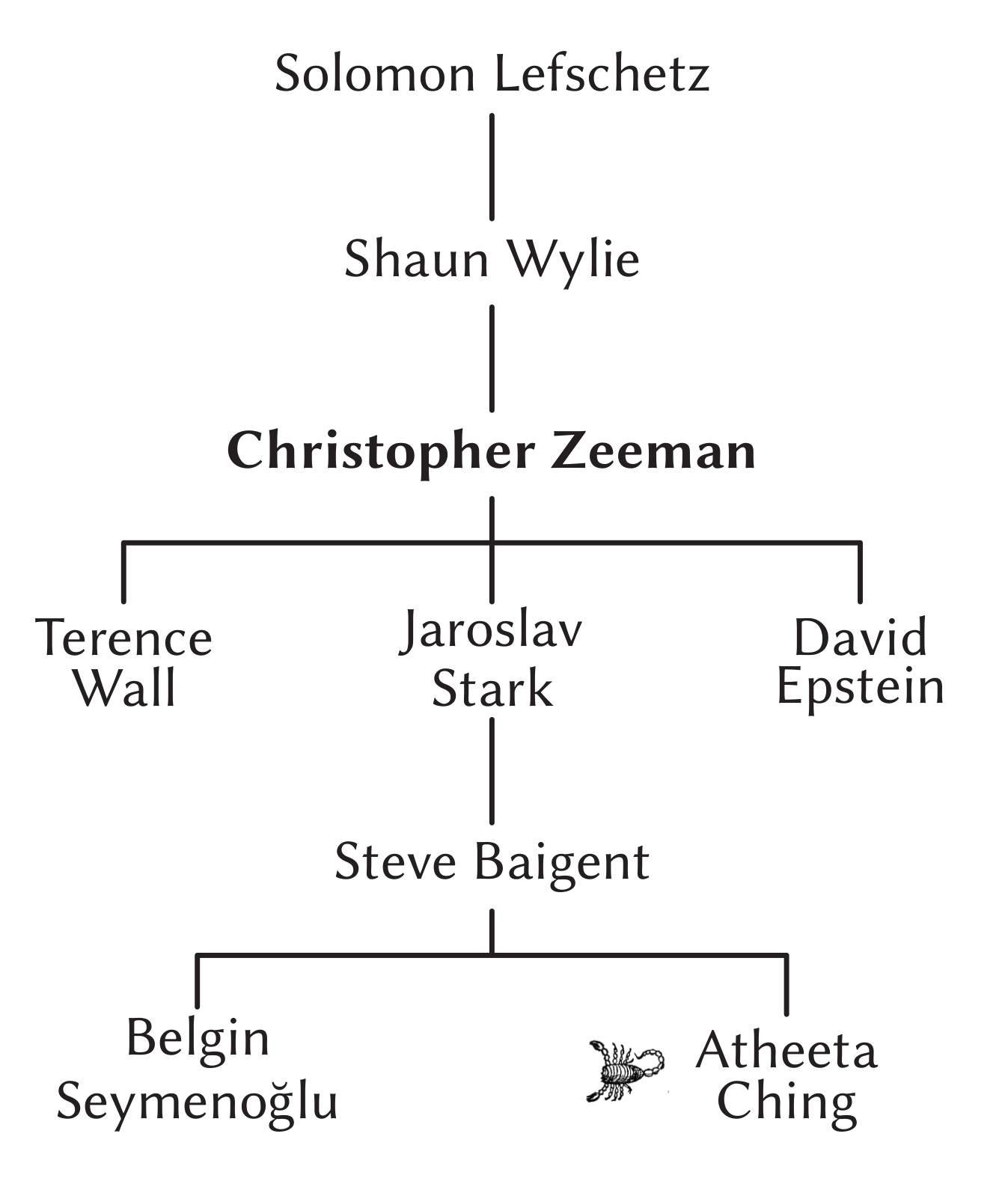Part of Zeeman’s (academic) family tree

In total, Zeeman had 29 PhD students, including David Epstein, Terry Wall and Jaroslav Stark, and over 700 other descendants, including me!

It is now about two years since Zeeman passed away aged 91, on 23 February 2016. I never met him in person, but I have seen and felt the effects of his legacy, and am proud to be (academically!) descended from him. Some of his methods in dynamical systems which he applied in mathematical ecology came in handy for my research in population genetics.

Now I want to share my love of mathematics with the rest of the world, like Zeeman did, and there is no better place to start than Chalkdust. If you’re equally inspired, you should write for Chalkdust too! I look forward to reading it.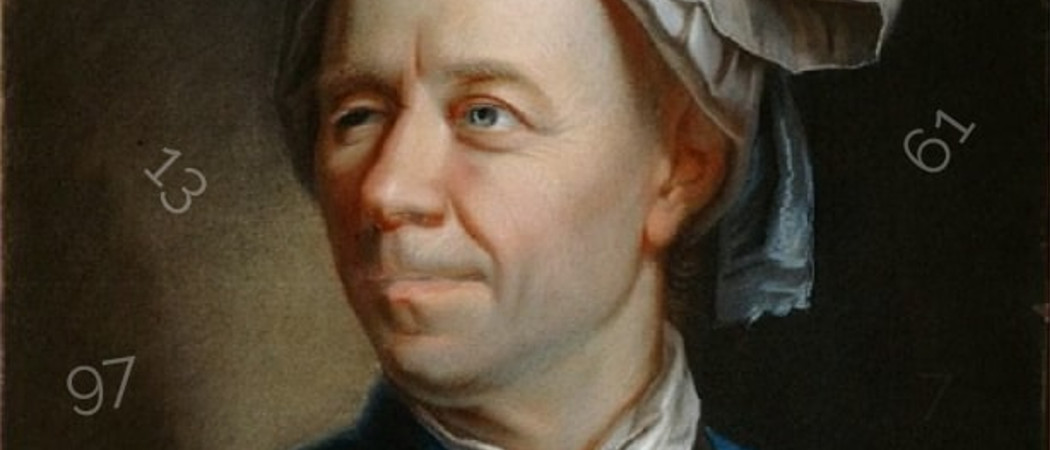# Primes à la Euler

Anyone with a more than a passing interest in numbers knows that there are infinitely many primes.If we highlight them in a grid like this, it is clear that the last digit of any prime must be either 1, 3, 7 or 9 (except for 2 and 5 of course). But there seems to be no good reason for primes to prefer any one of these four possibilities over any other. It is natural therefore to conjecture that

There are infinitely many primes whose last digit is $b$ for $b$ equal to any of 1, 3, 7, or 9.

Unless you have seen this before you probably don’t know how to prove it. When stuck trying to prove something and you don’t know what to do, it is often a good tactic to first try to prove something simpler than, but related to, what you really want to prove.

One way to simplify this particular problem is to notice the following: besides the exceptions 2 and 5, when we divide a prime $p$ by 10 there are four possibilities for the remainder, 1, 3, 7 or 9, which we write as $p \equiv 1, 3, 7 \text{ or } 9 \text{ (mod } 10 ).$

When we divide an odd prime by 4 though, there are only two possibilities, $1$ or $3$. So we might start by trying to prove that there are infinitely many primes $p$ such that $p \equiv b \text{ (mod 4)}$ for any $b$ equal to 1 or 3. This just means that the remainder when we divide $p$ by 4 is $b$. Let’s now see how Euler might have thought about this problem by looking at how he proved that there are infinitely many primes.

## Primes, infinite series and π

$$\sum_{n=1}^{\infty}\frac{1}{n} = 1 + \frac{1}{2} + \frac{1}{3} + \frac{1}{4} + \frac{1}{5} + \cdots = \prod_{\text{ primes } p }\frac{1}{1-\frac{1}{p}}.$$
Knowing that the left hand side diverges to $\infty$, we see immediately that the right hand side cannot be a finite product. And that’s it! The right side cannot be a finite product–so there are infinitely many primes! That’s Euler’s proof. But how do you prove the identity? Simple. Expand each factor of the right hand side into a geometric sum to get
$$\prod_{p}\left(1 + \frac{1}{p} + \frac{1}{p\hspace{1pt}^2} + \frac{1}{p\hspace{1pt}^3} \cdots \right).$$
Multiplying out the product involves choosing a $1/p\hspace{1pt}^k$ from each factor, multiplying these $1/p\hspace{1pt}^k$ together and summing over all the ways of choosing the value of $k$. The only resulting terms which are non-zero are those in which we pick $k$ to be non-zero for only finitely many primes and $k=0$ for all other primes. Doing so, we get $1/n$ for each positive integer $n$ precisely once, just as we wanted. This is a consequence of the fundamental theorem of arithmetic which says that each integer can be written as a product of primes in a unique way (up to the order of the primes). It is well worth thinking this through so that it makes sense because it is the most crucial part of the argument.

Euler used his product formula to prove even more. By taking the logarithm of $\infty$ to get $\infty$ and converting the infinite product into an infinite sum (this is not rigorous mathematics by today’s standards but Euler wasn’t one to let pedantic details spoil a beautiful argument!)
$$\infty = \log \left(\prod_{\text{ primes } p }\frac{1}{1-\frac{1}{p}} \right) = – \sum_{p}\log\left(1-\frac{1}{p} \right).$$
After expanding log using the power series
$$-\log(1-x) = x + \frac{x^2}{2} + \frac{x^3}{3} + \frac{x^4}{4} + \cdots,$$
valid for all $-1<x<1$, Euler gets
$$\infty = \sum_{p}\frac{1}{p} + \frac{1}{2}\sum_{p}\frac{1}{p\hspace{1pt}^2} + \frac{1}{3}\sum_{p}\frac{1}{p\hspace{1pt}^3} + \cdots.$$
Most of these sums are bounded. In fact,
$$\frac{1}{2}\sum_{p}\frac{1}{p\hspace{1pt}^2} + \frac{1}{3}\sum_{p}\frac{1}{p\hspace{1pt}^3} + \cdots < \sum_{p} \sum_{n = 2}^{\infty}\frac{1}{p\hspace{1pt}^n} = \sum_{p}\frac{1}{p\hspace{1pt}(p-1)} < \sum_{n=1}^{\infty}\frac{1}{n\hspace{1pt}^2} < \infty.$$
So we have the astonishing conclusion that
$$\sum_{p}\frac{1}{p} = \infty.$$
Remember that we would like to show that the primes are in some sense split evenly between the two classes 1 (mod 4) and 3 (mod 4) – and in particular that there are infinitely many of each type. Inspired by Euler’s argument involving infinite series, we next introduce the following alternating series
$$A = -\frac{1}{3} + \frac{1}{5} – \frac{1}{7} – \frac{1}{11} + \frac{1}{13} – \frac{1}{17} + \ldots =\sum_{p \neq 2 }\frac{(-1)^{(p-1)/2}}{p}$$
which has a plus sign in front of those primes which are 1 (mod 4) and a minus sign in front of those primes which are 3 (mod 4). The prime 2 doesn’t really feel like it belongs in this series and in particular it is not clear what the sign for $1/2$ ought to be. To avoid having to make an arbitrary choice we will just not include the prime 2.A pencil showing the largest `left-truncatable’ prime, created by Maths Inspiration. Image: Luciano Rila

Our strategy then is to prove that the series $A$ converges. If we could, it would then follow immediately from $\sum{1/p} = \infty$ that there are infinitely many primes of each type, 1 and 3 mod 4. Because if there were only finitely many of one of these types, the series $A$ would diverge.

Taking a cue from Euler’s original argument, we can add in the prime powers without changing whether or not the series converges (because, as we have seen, the sum of the reciprocals of the prime powers converges). So expanding the logarithm into an infinite series, it follows just as before that $A$ converges if and only if
$$-\sum_{p \neq 2}\log\left(1-\frac{(-1)^{(p-1)/2}}{p}\right)$$
converges. Exponentiating, this converges if and only if
$$\prod_{p \neq 2}\frac{1}{1-\frac{(-1)^{(p-1)/2}}{p}}$$
converges to something non-zero. We can expand this infinite product much like before by first writing each factor as a geometric sum
$$\prod_{p \neq 2}\left(1+ \frac{(-1)^{(p-1)/2}}{p} + \frac{1}{p\hspace{1pt}^2} + \frac{(-1)^{(p-1)/2}}{p\hspace{1pt}^3} + \cdots \right).$$
Expanding the product is a bit harder this time but not too bad. It is
$$\prod_{p \neq 2}\left(1+ \frac{(-1)^{(p-1)/2}}{p} + \frac{1}{p\hspace{1pt}^2} + \frac{(-1)^{(p-1)/2}}{p\hspace{1pt}^3} + \cdots \right) = 1 – \frac{1}{3} + \frac{1}{5} – \frac{1}{7} + \frac{1}{9} – \frac{1}{11} + \cdots$$
now with only odd terms and every other term having a minus sign. This one is more difficult because we need to keep track of all the minus signs. Multiplying out the product, we get $\pm1/n$ for each odd integer $n$ exactly once for some choice of sign. There are no even integers as we have excluded the prime 2. An integer appears with a minus sign if and only if it has an odd number of prime factors which are $3 \text{ (mod 4)}$. This is exactly the right hand side though since such integers are exactly those which are themselves $3 \text{ (mod 4)}$. You might want to stop to check this last point makes sense.

But wait. We recognise this (don’t we) as Leibniz’s famous series
$$\frac{\mathrm{\pi}}{4} = 1 – \frac{1}{3} + \frac{1}{5} – \frac{1}{7} + \frac{1}{9} – \frac{1}{11} + \cdots$$
which is certainly finite.

So, there we have it
$$\sum_{p \neq 2}\frac{(-1)^{(p-1)/2}}{p} = \text{“something finite”}$$
and we proved above that
$$\sum_{p\equiv 1 \text{ (mod 4)}}\frac{1}{p} + \sum_{p\equiv 3 \text{ (mod 4)}}\frac{1}{p} = \infty$$
because leaving out the prime 2 doesn’t change whether or not the series converges.
By adding and subtracting these two equations we conclude that.
$$\sum_{p\equiv 1 \text{ (mod 4)}}\frac{1}{p} = \infty \:\:\:\:\:\: \text{ and } \:\:\:\:\:\: \sum_{p\equiv 3 \text{ (mod 4)}}\frac{1}{p} = \infty.$$
What an astounding thing to follow from an infinite series for $\mathrm{\pi}$!

## The proof

As pleased as we are with this dramatic conclusion, it doesn’t solve our original problem. We wanted to show that for each $b=$ 1, 3, 7, 9, there are infinitely many primes whose last digit is $b$. We can rewrite this condition by noticing the following equivalences which hold for all odd numbers $p$,
\begin{align*}
p \equiv 1 \text{ (mod 10)} &\Leftrightarrow p \equiv 1 \text{ (mod 5)} \\
p \equiv 3 \text{ (mod 10)} &\Leftrightarrow p \equiv 3 \text{ (mod 5)} \\
p \equiv 7 \text{ (mod 10)} &\Leftrightarrow p \equiv 2 \text{ (mod 5)} \\
p \equiv 9 \text{ (mod 10)} &\Leftrightarrow p \equiv 4 \text{ (mod 5)}.
\end{align*}

Convince yourself this is true by trying a few examples. So can we do for 5 what we just did for 4? It will be a bit harder this time because there are 4 remainder options for our primes rather than just two. Let’s first take some time to reflect on what made the argument work before we try to generalise it.

1. We had an infinite convergent series that factored into primes and could somehow distinguish between primes in different remainder classes modulo 4.
2. We took logarithms and “solved for” the two sums $\sum_{p \equiv 1 \text{ (mod 4)}}1/p$ and $\sum_{p \equiv 3 \text{ (mod 4)}}1/p$ by taking a linear combination with the logarithm of Euler’s product formula.
3. To deduce that these sums over primes were infinite, it was important that the original series not only converged, but that it converged to something non-zero. We needed it to be non-zero so we could take the logarithm and get a finite answer. It wasn’t important what the answer was, just that it was finite.

Point 1 suggests (or rather, it suggested to Dirichlet) that we assign coefficients $\chi(n)$ to each integer $n$ which only depend on the remainder of $n$ when divided by 5. These coefficients are to be used to detect which class the primes belongs to. They should also give rise to a nice convergent series which can be factored into a product over all primes
$$\sum_{n = 1}^{\infty}\frac{\chi(n)}{n} = \prod_{p}\text{factor(p)}.$$
We will be able to factor the series just as before if the coefficients have the special property
$$\chi(mn) = \chi(m)\chi(n) \text{ for all positive integers } m \text{ and } n.$$
If $\chi$ has this special property then we can write
$$\sum_{n=1}^{\infty}\frac{\chi(n)}{n} = \prod_{p}\frac{1}{1-\frac{\chi(p)}{p}}.$$
The proof is almost exactly the same as before. This multiplicativity property is quite restrictive, especially if we also require $\chi(n)$ to only depend on the remainder of $n$ by 5. First of all, if we don’t want our $\chi(n)$ to equal zero for all $n$ then we must have $\chi(1) = 1$ since $\chi(n) =\chi(n\cdot1) = \chi(n)\cdot \chi(1)$ so $\chi(1) \neq 1$ implies $\chi(n) = 0$ for all $n$. If we set $\chi(2) = \alpha$, say, $\chi(4)$ is determined to be $\chi(4)=\chi(2\cdot2) = \chi(2) \cdot \chi(2) = \alpha^2$. Similarly, the value of $\chi(3)$ is determined by $\chi(3) = \chi(4\cdot2) = \chi(4) \cdot \chi(2) = \alpha^3$. Here we used that $4\cdot 2 = 8 \equiv 3\text{(mod 5)}$. So we just need to decide on a value for $\alpha$ and $\chi(0)$. Recalling what happened before, we will set $\chi(0)$ to be zero. 5 is the only prime in this remainder class anyway so if the point of these coefficients is to detect primes, they ought to be zero on this class. We don’t actually have much choice for $\alpha$ since $1 = \chi(1)= \chi(3\cdot 2) = \chi(3) \cdot \chi(2) = \alpha^4$. Therefore, $\alpha$ must equal $1$, $i$, $-1$ or $-i$. Each of these choices of $\alpha$ gives a different $\chi$ which we will denote $\chi_\alpha$. In table format:Each $\chi$ gives us a different series
\begin{align*}
\sum_{n=1}^{\infty}\frac{\chi_1(n)}{n} &= \frac{1}{1} + \frac{1}{2} + \frac{1}{3} + \frac{1}{4} + \frac{1}{6} + \frac{1}{7} + \frac{1}{8} + \frac{1}{9} + \cdots\\
\sum_{n=1}^{\infty}\frac{\chi_{i}(n)}{n} &= \frac{1}{1} + \frac{i}{2} -\frac{1}{3} – \frac{i}{4} + \frac{1}{6} + \frac{i}{7} – \frac{1}{8} – \frac{i}{9} + \cdots\\
\sum_{n=1}^{\infty}\frac{\chi_{-1}(n)}{n} &= \frac{1}{1} – \frac{1}{2} – \frac{1}{3} + \frac{1}{4} + \frac{1}{6} – \frac{1}{7} – \frac{1}{8} + \frac{1}{9} + \cdots\\
\sum_{n=1}^{\infty}\frac{\chi_{-i}(n)}{n} &= \frac{1}{1} – \frac{i}{2} + \frac{i}{3} – \frac{1}{4} + \frac{1}{6} – \frac{i}{7} + \frac{i}{8} – \frac{1}{9} + \cdots
\end{align*}

This is nice because point 2 above suggests that we need \emph{four} series to solve for our \emph{four} unknowns $\sum_{p \equiv b \text{ (mod 5)}}1/p$ with $b = 1,2,3$ or 4. Let us look at each of these four series in turn and see if they have the properties we want.

Case 1: $\alpha = 1$. This one diverges and is the one that tells us that $\sum_{p}1/p = \infty$. In some sense this is the most important one because it is the one that tells us we have infinitely many primes when we include all remainder classes.

Case 2: $\alpha = i$. This one involves the complex number $i$ and it is natural to look at its real and imaginary parts separately. Remember that we want to show that it converges to a finite non-zero number but we are not overly interested in what that number is, so long as it isn’t 0 or $\infty$. This will happen provided the real and imaginary parts both converge to some finite limits that are not both zero. This in turn is fairly easy to prove by grouping the positive and negative terms together. We will do the real part and invite the reader to fill in the details for the imaginary part.
\begin{align*}
\frac{1}{1} -\frac{1}{3} + \frac{1}{6} – \frac{1}{8} + \frac{1}{11} – \frac{1}{13}+\cdots &= \sum_{k = 1}^{\infty}\left(\frac{1}{5k – 4} – \frac{1}{5k-2}\right) \\
&= \sum_{k=1}^{\infty}\frac{2}{(5k-4)(5k-2)} < \sum_{n=1}^{\infty}\frac{2}{n\hspace{1pt}^2} < \infty.
\end{align*}
Notice that each term $\frac{2}{(5k – 4)(5k-2)}$ is positive so the sum is certainly not zero.

Case 3: $\alpha = -1$. We can do this by collecting the terms into groups of 4 and using a little algebra.
\begin{align*}
\frac{1}{1} &-\frac{1}{2} – \frac{1}{3} + \frac{1}{4} + \frac{1}{6} – \frac{1}{7} – \frac{1}{8} + \frac{1}{9} + \cdots = \sum_{k=1}^{\infty}\left( \frac{1}{5k-4}-\frac{1}{5k-3}-\frac{1}{5k-2}+\frac{1}{5k-1} \right) \\
&= \sum_{k=1}^{\infty}\tfrac{(5k-3)(5k-2)(5k-1)-(5k-4)(5k-2)(5k-1)-(5k-4)(5k-3)(5k-1)+(5k-3)(5k-2)(5k-1)}{(5k-4)(5k-3)(5k-2)(5k-1)} \\
&= \sum_{k=1}^{\infty}\frac{20k – 2}{(5k-4)(5k-3)(5k-2)(5k-1)} < \infty.
\end{align*}
Again, each term $\frac{20k – 2}{(5k-4)(5k-3)(5k-2)(5k-1)}$ is positive so whatever this sum is, it is positive.

Case 4: $\alpha =-i$. This one is very similar to case 2 so we will leave the details to the interested reader to fill in for herself.

Now that we know our series converge to non-zero limits we can take logarithms to convert our products over primes into sums over primes. Expanding log into an infinite series and bounding the terms involving prime powers exactly as before we get the following four equations:

$$\sum_{p}\frac{\chi_1(p)}{p} = \infty, \:\:\: \sum_{p}\frac{\chi_i(p)}{p} = \text{“finite”}, \:\:\: \sum_{p}\frac{\chi_{-1}(p)}{p} = \text{“finite”}, \:\:\: \sum_{p}\frac{\chi_{-i}(p)}{p} = \text{“finite”},$$
which we can write more explicitly as
\begin{align*}
\lim_{n \rightarrow \infty}\sum_{\substack{ p \leq n \\ p \equiv 1\text{ (mod 5)}}}\frac{1}{p} + \sum_{\substack{ p \leq n \\ p \equiv 2\text{ (mod 5)}}}\frac{1}{p} + \sum_{\substack{ p \leq n \\ p \equiv 3\text{ (mod 5)}}}\frac{1}{p} + \sum_{\substack{ p \leq n \\ p \equiv 4\text{ (mod 5)}}}\frac{1}{p} &= \infty \\
\lim_{n \rightarrow \infty}\sum_{\substack{ p \leq n \\ p \equiv 1\text{ (mod 5)}}}\frac{1}{p} + \sum_{\substack{ p \leq n \\ p \equiv 2\text{ (mod 5)}}}\frac{i}{p} – \sum_{\substack{ p \leq n \\ p \equiv 3\text{ (mod 5)}}}\frac{i}{p} – \sum_{\substack{ p \leq n \\ p \equiv 4\text{ (mod 5)}}}\frac{1}{p} &= \text{“finite”} \\
\lim_{n \rightarrow \infty}\sum_{\substack{ p \leq n \\ p \equiv 1\text{ (mod 5)}}}\frac{1}{p} – \sum_{\substack{ p \leq n \\ p \equiv 2\text{ (mod 5)}}}\frac{1}{p} – \sum_{\substack{ p \leq n \\ p \equiv 3\text{ (mod 5)}}}\frac{1}{p} + \sum_{\substack{ p \leq n \\ p \equiv 4\text{ (mod 5)}}}\frac{1}{p} &= \text{“finite”} \\
\lim_{n \rightarrow \infty}\sum_{\substack{ p \leq n \\ p \equiv 1\text{ (mod 5)}}}\frac{1}{p} – \sum_{\substack{ p \leq n \\ p \equiv 2\text{ (mod 5)}}}\frac{i}{p} + \sum_{\substack{ p \leq n \\ p \equiv 3\text{ (mod 5)}}}\frac{i}{p} – \sum_{\substack{ p \leq n \\ p \equiv 4\text{ (mod 5)}}}\frac{1}{p} &= \text{“finite”}.
\end{align*}
That’s a bit messy so let’s introduce the notation $S_b(n) = \sum_{\substack{ p \leq n \\ p \equiv b \text{ (mod 5)}}}1/p$ for $b = 1, 2, 3, 4$ and rewrite it as
\begin{align*}
\lim_{n \rightarrow \infty} S_1(n) + S_2(n) + S_3(n) + S_4(n) &= \infty \\
\lim_{n \rightarrow \infty} S_1(n) + i S_2(n) -i S_3(n) – S_4(n) &= \text{“finite”} \\
\lim_{n \rightarrow \infty} S_1(n) – S_2(n) – S_3(n) + S_4(n) &= \text{“finite”} \\
\lim_{n \rightarrow \infty} S_1(n) -i S_2(n) +i S_3(n) – S_4(n) &= \text{“finite”}.
\end{align*}
This looks like a simple system of four linear equations in four unknowns, but it involves limits and infinity and one must not be tempted into taking the limits too soon and writing nonsense like $\infty +i \infty -i\infty -\infty = \text{“finite”}$. We leave the task of carefully deducing that $\sum_{p\equiv b \text{ (mod 5)}}1/p = \lim_{n \rightarrow \infty} S_b(n) = \infty$ for each $b = 1, 2, 3, 4$ as an exercise. If successful, the reader will have finished the proof of our original conjecture, and proved that there are infinitely many primes ending in each of 1, 3, 7, and 9!